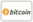Bookcover of Integral Equations and Transforms
Booktitle:

# Integral Equations and Transforms

Scholars' Press (2016-07-11 )eligible for voucher
ISBN-13:

### 978-3-659-84047-0

ISBN-10:
3659840475
EAN:
9783659840470
Book language:
English
Blurb/Shorttext:
The book entitled Integral equation and Transforms consists of eight chapters.This book contains preliminary concepts of integral equations, some problems which give rise to integral equations, conversion of ordinary differential equations into integral equations, classification of linear integral equations, integro-differential equations. Fredholm equations, degenerate kernels, Hermitian and symmetric kernels, the Hilbert-Schmidt theorem, Hermitization and symmetrization of kernels, solution of integral equations with green’s function type kernels,types of Voltera equations, Resolvent kernel of Voltera equations, convolution type kernels,non- linear Voltera equations. Fourier integral equations, Laplace integral equations Hilbert transform, Fourier integral theorem, Fourier transform Fourier cosine and sine transform,the convolution integral, multiple Fourier transform, solution of partial differential equation by means of Fourier transform, the Laplace transform of some elementary functions, Laplace transform of derivatives, the convolution of two functions, inverse formula for Laplace transform and solutions of ordinary differential equations by Laplace transform.
Publishing house:
Scholars' Press
Website:
http://www.scholars-press.com
By (author) :
Number of pages:
464
Published at:
2016-07-11
Stock:
Available
Category:
Mathematics
Price:
114.90 €
Keywords:
Integral transform, Integral Equation, Mathematical Method.### Categories